American Journal of Theoretical and Applied Statistics
Volume 4, Issue 6, November 2015, Pages: 630-639

Significance Test in Meta-analysis Approach: A theoretical Review

Habib Ahmed Elsayir

Department of Mathematics, Al Qunfudha University College, Umm Al Qura University, Al Qunfudha, Saudi Arabia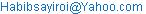Habib Ahmed Elsayir. Significance Test in Meta-analysis Approach: A theoretical Review. American Journal of Theoretical and Applied Statistics. Vol. 4, No. 6, 2015, pp. 630-639. doi: 10.11648/j.ajtas.20150406.35

Abstract: Meta-analysis, a statistical procedure that integrates the results of several independent studies, plays a central role in statistical research, and a very important task in research problems and statistical significance tests. This paper discusses these principles, along with the practical steps in performing meta-analysis. It describes the issue of meta-analysis, explains what meta-analysis is, how it is done and how it can be interpreted. Some related problems such as statistical significance, effect size and power analysis are described. Examples of implementation on theoretical data would be carried. Results, conclusions, recommendations on the use of meta-analysis would be summarized.

Keywords: Effect Size, Meta-analysis, Sample Size, Sensitivity Analysis, Significance Test, Systematic Review

1. Introduction

Meta-analysis used in many application fields. Pharmaceutical companies use meta-analysis to gain approval for new drugs. Clinicians and applied researchers in medicine, education, psychology, criminal justice, and several of other fields use meta-analysis to determine which interventions work, and which ones work best. Meta-analysis is also widely used in basic research to evaluate the evidence in areas as diverse as sociology, social psychology, sex differences, finance and economics, political science, marketing, ecology and genetics, among others. Decisions about the utility of an intervention or the validity of a hypothesis cannot be based on the results of a single study, since results vary from one study to another. Rather, a mechanism is needed to synthesize data across studies. Narrative reviews had been used for this purpose, but considered largely subjective (different conclusions) and becomes impossibly difficult when there are more than a few studies involved. Meta-analysis, by contrast, applies objective formulas and can be used with any number of studies.

Meta-analysis is a statistical procedure that integrates the results of several independent studies considered to be "combinable". Well conducted meta-analyses allow a more objective appraisal of the evidence than traditional narrative reviews, provide a more precise estimate of a treatment effect, and may explain heterogeneity between the results of individual studies. Conducted meta-analyses, on the other hand, may be biased owing to exclusion of relevant studies or inclusion of inadequate studies Egger, M et al (1997). It is is a statistical technique in which the results of two or more studies are mathematically combined to see if the overall effect is significant in order to improve the reliability of the results.

When there are multiple studies with conflicting results, meta-analysis will be useful since it combines and tests the results of all the studies. The result is the same as doing one study with a really big sample size, one large enough to conclusively demonstrate an effect if there is one, or conclusively reject an effect if there isn't one of an appreciable size John H. McDonald (2014).

Studies chosen for inclusion in a meta-analysis must be sufficiently similar in a number of characteristics in order to accurately combine their results. When the treatment effect (or effect size) is consistent from one study to the next, meta-analysis can be used to identify this common effect. When the effect varies from one study to the next, meta-analysis may be used to identify the reason for the variation. statistical-solutions-software

In this article, the general steps involved in doing a meta-analysis will be described. Some of the basic steps of a meta-analysis will be explained. Sufficient detail can be seen in: Berman and Parker (2002), Gurevitch and Hedges (2001), Hedges and Olkin (1985), or some other books. This paper also gives a brief demonstration of basic methodologies of effect size, reviews issues of the topic, accompanied by numerical illustrations. Tables made are computed from different sources and verified using online software on effect size (see the list of websites references here). The use of effect sizes, however, has generally been limited to meta-analysis for combining and comparing estimates from different studies. This is despite the fact that measures of effect size have been available for decades, Huberty (2002).

The concept of effect size is tight to a school of methodology which known as meta-analysis, (see Baker, R. & Dwyer, F. (2000), Biostat (2006), Poston, J. M., & Hanson, W. E. (2010). Heavily laying on Rosenthal (1994), Rosenthal & Rosnow (2000), has introduced a useful summary of effect sizes computation and transformations for inferential statistics. Michael Fur (2008) has also discussed effect sizes and their links to inferential statistics.

Meta analysis always deals with two issues: publication bias (also known as the file drawer problem) and the varying quality of the studies. Publication bias is "the systematic error introduced in a statistical inference by conditioning on publication status. Publication bias can lead to misleading results when a statistical analysis is performed after assembling all of the published literature on some subject. Gerard E. Dallal (2015).

Meta-analysis would be used for the following purposes:

1)  To establish statistical significance with studies that has conflicting results.

2)  To develop a more correct estimate of effect magnitude.

3)  To provide a more complex analysis of harms, safety data, and benefits.

4)  To examine subgroups with individual numbers that are not statistically significant.

There is, as yet, no unanimously accepted strategy for performing a meta-analysis but researchers agree that each meta-analysis should be conducted like a scientific experiment and begin with a protocol, which clearly states its aim and methodology. J Hypertens (1996). Meta-analysis should be as carefully planned as any other research project, with a detailed written protocol being prepared in advance. Egger, M. et al (1997).

Potential advantages of meta-analysis (eg. over classical literature reviews, simple overall means of effect sizes etc.) include:(see Jonathan J Deeks, Julian PT Higgins and Douglas G Altman, and see. statistical-solutions-software webpage/)

1)  Derivation and statistical testing of overall factors / effect size parameters in related studies

2)  The ability to answer questions not posed by individual studies and generalization to the population of studies.

3)  Ability to control for between-study variation.

4)  Including moderators to explain variation.

5)  Higher statistical power to detect an effect than in ‘n=1 sized study sample’

6)  An improvement in precision.

Considered an evidence-based resource, meta-analysis offers the opportunity to critically evaluate and statistically combine results of comparable studies or trials. However, disadvantage of meta-analysis is that - (see The Himmelfarb Health Sciences Library (2011)-it looks difficult and time consuming to identify appropriate studies and not all studies provide adequate data for inclusion and analysis. In addition to that it requires advanced statistical techniques as well as the issue of heterogeneity of study populations.

In general, Weaknesses of Meta Analysis is as follows: (see the statistical-solutions-software web page).

1)  Meta-analysis can never follow the rules of hard science. Weaknesses include:

2)  Sources of bias are not controlled by the method.

3)  A good meta-analysis of badly designed studies will still result in bad statistics.

4)  Heavy reliance on published studies, which may create exaggerated outcomes, as it is very hard to publish studies that show no significant results. (File Drawer Problem).

5)  Dangers of Agenda Driven Bias: From an integrity perspective, researchers with a bias should avoid meta-analysis and use a less abuse-prone (or independent) form of research.

A meta-analysis answers three general questions: (see Overview of Meta-Analysis web page):

1)  Central tendency – The central purpose of a meta analysis is to test the relationship between two variables such that X affects Y. Central tendency identifies whether X affects Y via statistically summarizing significance levels, effect sizes, and/or confidence intervals, and try to answer whether X affects Y, is the effect significant, and how strong is that effect?

2)  Variability – There is always some degree of variation between the outcomes of the individual studies that compose the meta-analysis. The question is whether the degree of variability is significantly different than what we would expect by chance alone. If so, then its called heterogeneity.

3)  Prediction – If there is heterogeneity (variability), then we look for moderating variables that explain the variability (does the effect of X on Y differ with moderator variables?).

1.1. Meta-Analysis Basic Steps

There are generally five separate steps in conducting a meta-analysis: (see Meta-analysis. From PsychWiki web page).

1.  Hypothesis defining – A well-defined statement of the relationship between the variables under investigation must be determined to define carefully the inclusion and exclusion criteria when locating potential studies.

2.  Locate the studies – A meta-analysis is only informative if it adequately summarizes the existing literature, such as database searches, unpublished studies, conference proceedings, etc).

3.  Data collection– Gather empirical findings from primary studies (e. g., p-value, effect size, etc) and input into statistical database.

4.  Effect sizes Calculation– Calculate the overall effect by converting all statistics to a common metric, making adjustments as necessary to correct for issues like sample-size or bias, and then calculating central tendency (e. g., mean effect size and confidence intervals around that effect size) and variability (e. g., heterogeneity analysis).

5.  Variables Analysis – If heterogeneity exists, you may want to analyze moderating variables by coding each variable in the database and analyzing either mean differences (for categorical variables) or weighted regression (for continuous variables) to see if the variable accounts for the variability in the effect size.

1.2. Steps of Conducting a Meta-analysis

First, select suitable statistical approach:

Generally, there are three different statistical approaches to conduct a meta-analysis so first you need to choose which approach best fits your needs. Detailed comparison of these three approaches, are found in (Johnson, Mullen, & Salas, 1995) and (Schmidt and Hunter, 1999).

Hedges & Olkin Approach – see (Hedges, 1981); (Hedges, 1982); (Hedges & Olkin, 1985)

1.  Rosenthal & Rubin Approach – see (Rosenthal, 1991); (Rosenthal & Rubin, 1978); (Rosenthal & Rubin, 1988)

2.  Hunter, Schmidt, & Jackson - see (Hunter, Schmidt, & Jackson, 1982); (Hunter & Schmidt, 1990)

Second, choose which effect size index to calculate:

The commonly used effect size indexes are "the "r" family and the "d" family" of effect sizes. Since "r" and "d" can be transformed into each other statistically you may wonder why it matters which metric you choose. Empirical research can take many forms (e. g., dichotomous and/or continuous, dichotomous and/or continuous, two variables relationships, etc) and the form of research you are analyzing helps determine which metric may be best to use. For complete information and statistical formulas for all effect size indexes for each form of research, see (Lipsey & Wilson (2001), (Practical Meta-Analysis).

1.  The r family – Correlation Coefficient - The "r" family includes all types of correlation coefficients (e. g., r, phi, rho, etc) and (Johnson & Eagly, (2000) suggest using r when the studies composing the meta analysis primarily report the correlation between variables, but also see (Rosenthal & DiMatteo, (2001) for a discussion of the advantages of using r over d.

2.  The d family – Standardized Difference - The "d" family includes Cohen's d (unweighted) and Hedges g (weighted), and (Johnson & Eagly, (2000) suggest using d when the studies composing the meta-analysis primarily report ANOVAs and t-tests comparisons between groups.

There are two basic options -- use specialized software designed to conduct meta-analyses, or use standard statistical software such as SPSS and SAS. For websites provide effect size calculations and software see (Becker, L., (2000), Biostat (2006), Buchner, A, and Karl L. Wuensch (2010)).

1.  SPSS and SAS.

2.  The David B. Wilson website provides an excel spreadsheet for calculating effect sizes, and SPSS and SAS. MIX 2.0. MIX 2.0 - Professional Software for Meta-analysis in Excel. Meta-Analysis. Developed by (Schwarzer, 1996), it can be found on the Ralf Schwarzer website and each of the three meta-analytic approaches can be selected (i. e., Hedges/Olkin approach, Rosenthal approach, or Hunter/Schmidt/Jackson approach).

3.  META (Meta-Analysis Easy to Answer). Developed by David A. Kenny, a description of the software can be found on the David A. Kenny website.

4.  Meta-Analysis Calculator. Developed by Larry C. Lyons as a web based meta-analysis application and companion to the meta-analysis Pages.

5.  CMA (Comprehensive Meta-Analysis). Developed by many of the experts in meta-analyses, it includes a comparison between CMA and other meta-analytic software.

2. The Meta-analysis Procedure

The basic idea of a meta-analysis is that you take a weighted average of the difference in means, slope of a regression, or other statistic across the different studies. Experiments with larger sample sizes get more weight, as do experiments with smaller standard deviations or higher r2 values John H. McDonald (2014). You can then test whether this common estimate is significantly different from zero.

Before starting collecting studies, it's essential to decide which ones are to be included ore excluded through objective criteria. For instance, if you're looking at the effects of a drug on a disease, you might decide that only double-blind, placebo-controlled studies are worth looking at, or you might decide that single-blind studies are acceptable; or you might decide that any study at all on the drug and the disease should be included. Sample size shouldn't be used as a criterion for including or excluding studies, because the statistical techniques used for the meta-analysis will give studies with smaller sample sizes the lower weight they deserve. John H. McDonald (2014).

It is important to obtain all relevant studies, because loss of studies can lead to bias in the study. Typically, published papers and abstracts are identified by literature search. Crosschecking of references, citations in review papers, and communication with scientists who have been working in the relevant field are important methods used to provide a comprehensive search. A B Haidich. (2010).

It is not feasible to find absolutely every relevant study on a subject. Some or even many studies may not be published, and those that are might not be indexed in computer-searchable databases. The decision whether to include unpublished studies is difficult. Although language of publication can provide a difficulty, it is important to overcome this difficulty, provided that the populations studied are relevant to the hypothesis being tested. A B Haidich. (2010).

A critical issue in meta-analysis is what's known as the "file-drawer effect"; people who do a study and fail to find a significant result are less likely to publish it than if they find a significant result. To limit the file-drawer effect, it's important to do a thorough literature search, including really obscure journals, then try to see if there are unpublished experiments. To find out about unpublished experiments, you could look through summaries of funded grant proposals, which for government agencies; look through meeting abstracts in the appropriate field; write to the authors of published studies; and send out appeals on e-mail mailing lists. There are ways to estimate how many unpublished, non-significant studies there would have to be to make the overall effect in a meta-analysis non-significant. If that number is absurdly large, you can be more confident that your significant meta-analysis is not due to the file-drawer effect.

2.1. Systematic Review and Meta-analysis

A subset of systematic reviews; a method for systematically combining pertinent qualitative and quantitative study data from several selected studies to develop a single conclusion that has greater statistical power. This conclusion is statistically stronger than the analysis of any single study, due to increased numbers of subjects, greater diversity among subjects, or accumulated effects and results. Just like other research articles, can be of varying quality, systematic reviews answer a defined research question by collecting and summarizing all empirical evidence that fits pre-specified eligibility criteria. A meta-analysis is the use of statistical methods to summarize the results of these studies. There are some questions that must be asked when assessing the quality of a systematic review, such as: (see the web page of National Center for Biotechnology Information)

Was the review conducted according to a pr-specified protocol?

Were the "right" types of studies eligible for the review?

Was the method of identifying all relevant information comprehensive?

Was the data abstraction from each study appropriate?

How was the information synthesized and summarized?

The strength of a systematic review lies in the transparency of the process, allowing the reader to focus on the decision made in compiling the information, rather than a simple contrast of one study to another as sometimes occurs in other types of reviews. Well-conducted systematic review attempts to reduce the possibility of bias in the method of identifying and selecting studies for review. Mathematically combining data from a series of well-conducted primary studies may provide a more precise estimate of the underlying "true effect" than any individual study. In other words, by combining the samples of the individual studies, the size of the "overall sample" is increased, enhancing the statistical power of the analysis and reducing the size of the confidence interval for the point estimate of the effect. It is also more efficient to communicate a pooled summary than to describe the results for each of the individual studies.

For these reasons, a meta-analysis of similar, well-conducted, randomized, controlled trials has been considered one of the highest levels of evidence. When the existing studies have important scientific and methodological limitations, including smaller sized samples (which is more often the case), the systematic review may identify where gaps exist in the available literature. In this case, an exploratory meta-analysis can provide a plausible estimate of effect that can be tested in subsequent studies.

Conducting a meta-analysis does not overcome problems that were inherent in the design and execution of the primary studies. It also does not correct biases as a result of selective publication, whereby studies that report dramatic effects are more likely to be identified, summarized, and subsequently pooled in meta-analysis than studies that report smaller effect sizes (publication bias). Combining studies of poor quality with those that were more rigorously conducted may not be useful and can lead to worse estimates of the underlying truth or a false sense of precision around the truth. A false sense of precision may also arise when various subgroups of subjects defined by characteristics such as their age or gender differ in their observed response. In such cases, reporting an aggregate pooled effect might be misleading.

A sensitivity analysis is essential to assess the robustness of combined estimates to different assumptions and inclusion criteria. Egger, M. et al (1997). Opinions will often diverge on the correct method for performing a particular meta-analysis. The robustness of the findings to different assumptions should therefore always be examined in a thorough sensitivity analysis.

2.2. A Study Example

Seto KC, etal (2011) reviewed the English language literature for studies that monitor urban land-use change using satellite or airborne remotely sensed data published between 1988 and December 2008. In analysis, the study had to meet the following four criteria:

1.  Study must quantify the urban area extent for at least in one point in time.

2.  Study must quantify either the rate or amount of urban land expansion over a specific period of time.

3.  Study area extent must be at city, metro, or regional scale (<100,000 km).

4.  Study must not repeat the results presented in another paper.

The literature review generated more than 1,000 papers. Among these, filtered those that met criteria 1 and 2, which resulted in 264 papers, further narrowed this set of papers to those that meet criteria 3 and 4, which yielded 180 papers. In addition to this set of peer-reviewed papers, the authors reviewed and included a World Bank study that was similar in method and scientific rigor and used a multivariate regression on the pooled dataset to model the global rate of urban land expansion. They selected a range of independent variables based on urban theory and models, representing the major forces that drive the physical expansion of urban land cover. Dependent variable was a single annual rate for each decadal period in each study. Results showed considerable variation in the rates of urban expansion over the study period. Variations in urban expansion rates point to differences in national and regional socio-economic environments and political conditions.

2.3. Meta-analyses Evolution

The classical meta-analysis compares two treatments while network meta-analysis (or multiple treatment meta-analysis) can provide estimates of treatment of multiple treatment regimens. Meta-analysis can also be used to summarize the performance of diagnostic and prognostic tests. However, studies that evaluate the accuracy of tests have a unique design requiring different criteria to appropriately assess the quality of studies and the potential for bias.

Furthermore, there are many methodologies for advanced meta-analysis that have been developed to address specific concerns, such as multivariate meta-analysis. Meta-analysis is no longer a novelty in medicine. Numerous meta-analyses have been conducted for the same medical topic by different researchers. Recently, there is a trend to combine the results of different meta-analyses, known as a meta-epidemiological study, to assess the risk of bias.

3. Computing Effect Size in Meta-analysis

Methods used for meta-analysis use a weighted average of the results techniques to which can be broadly classified into two models Egger, M. et al (1997), the difference consisting in the way the variability of the results between the studies is treated. The "fixed effects" model considers that the variability is exclusively due to random variation. Therefore, if all the studies were infinitely large they would give identical results. The "random effects" model assumes a different underlying effect for each study and takes this into consideration as an additional source of variation, which leads to somewhat wider confidence intervals than the fixed effects model.

Some statisticians feel that other statistical approaches are more appropriate than either of the above. One approach uses Bayes's theorem. Bayesian statisticians express their belief about the size of an effect by specifying some prior probability distribution before seeing the data, and then they update that belief by deriving a posterior probability distribution, taking the data into account. Bayesian models are available under both the fixed and random effects assumption, but this approach is controversial because the definition of prior probability will often be based on subjective assessments and opinion. Egger, M. et al (1997).

Effect size is an important tool in reporting and interpreting effectiveness, and has many advantages over the use of tests of statistical significance. 'Effect size' is valuable for quantifying the effectiveness of a particular intervention, relative to some comparison, and a one of the tools that will help researchers move beyond null hypothesis testing. Effect size is a name given to a set of indices that measure the magnitude of a treatment effect. Unlike significance tests, these indices are independent of sample size. Effect size measures are the common currency of meta-analysis studies that summarize the findings from a specific area of research. Effect size quantifies the size of the difference between two groups, and may therefore be said to be a true measure of the significance of the difference. Another use of effect size is its use in performing power analysis, (see Buchner, A., Erdfelder, E. and Faul, F (2009). Researcher designers use power analysis to minimize the likelihood of both false positives and false negatives (Type I and Type II errors, respectively), Richard A. Zeller and Yan Yan (2007).

3.1. Effect Sizes & Confidence Intervals

Meta analysis shows findings in terms of effect sizes. The effect size provides information about how much change is evident across all studies and for subsets of studies. There are many different types of effect size, but they fall into two main types: standardized mean difference (e. g., Cohen's d or Hedges g) or correlation (e. g., Pearson's r). It is possible to convert one effect size into another, so each really just offers a differently scaled measure of the strength of an effect or a relationship.

The standardized mean effect size is basically computed as the difference score divided by the standard deviation of the scores.

In meta-analysis, effect sizes should also be reported with: The number of studies and the number of effects used to create the estimate. Confidence intervals to help readers determine the consistency and reliability of the mean estimated effect size. Tests of statistical significance can also be conducted and on the effect sizes. Different effect sizes are calculated for different constructs of interest, as predetermined by the researchers based on what issues are of interest in the research literature.

A number of statistics are sometimes proposed as alternative measures of effect size, other than the 'standardized mean difference'. One of these is the Proportion of variance accounted for, the R2 which represents the proportion of the variance in each that is 'accounted for' by the other. There are also effect size measures for multivariate outcomes. A detailed explanation can be found in Olejnik and Algina (2000). Calculating effect size is important when testing the goodness fit, or contingency test, for this test, the effect size symbol is w. Once effect size is known, this information can be used to calculate the number of participants needed and the critical chi-square value (for sample size rules (see Aguinis, H. & Harden, E. E. (2009)), (and see the effect of sample size on effect size in Slavin, R., & Smith, D. (2008).

The developed formulas for effect size calculation vary depending on whether the researcher plans to use analysis of variance (ANOVA), t test, regression or correlation, (see Morris and DeShon's (2002)). Formulas used to measure effect size can be computed in either a standardized difference between two means, or in the correlation between the independent variable classification and the individual scores on the dependent variable, which is called the "effect size correlation" (Rosnow & Rosenthal (1996).

Effect size for differences in means is given by Cohen's "d" Cohen, J. (1988), is defined in terms of population means (μs) and standard deviation (σ), as shown below: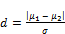(1)

There are several different ways that one could estimate σ from sample data which leads to multiple variants within the Cohen's d family.(see Karl L. Wuensch(2010)).

When using the root mean square standard deviation, the "d" is given as: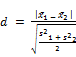(2)

A version of Cohen's d uses the pooled standard deviation and is also known as Hedges':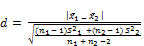(3)

The value can be obtained from an ANOVA program by taking the square root of the mean square error which is also known as the root mean square error.

Another model of Cohen's " d" using the standard deviation for the control group is also known as Glass' Δ (see Karl L. Wuensch (2010)), where: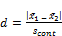(4)

The control group's standard deviation is used because it is not affected by the treatment. It is suggested to use a pooled within group standard deviation because it has less sampling error than the control group standard deviation such that equal size constrain is adopted. When there are more than two groups, the difference between the largest and smallest means divided by the square root of the mean square error will be used, i. e.: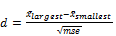(5)

As for OLS regression the measure of effects size is F which is defined by Cohen as follows: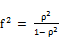(6)

Or, as usually computed by taking the square root of f2.

Once again there are several ways in which the effect size can be computed from sample data. It can be noted that η2 is another name for R2, the coefficient of determination, where: (see Karl L. Wuensch (2010)).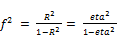(7)

The effect size used in analysis of variance is defined by the ratio of population standard deviations: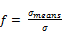(8)

Based on definitional formula in terms of population values, effect size w can be viewed as the square root of the standardized chi-square statistic.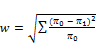(9)

And w is computed using sample data by the formula: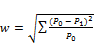(10)

According to Poston &Hanson(2010), when a study reports a hit rate (percentage of success after taking the treatment or no treatment), the following formula can be used:

d= arcsine(p1)+ arcsine(p2)

Where p1 and p2 are the hit rates of the two groups.

If the effect size estimate from the sample is d, then it is normally distributed, with standard deviation: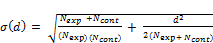(11)

(Whereand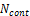are the numbers in the experimental and control groups, respectively.)

The control group will provide the best estimate of standard deviation, since it consists of a representative group of the population who have not been affected by the experimental intervention. Therefore, it is often better to use a 'pooled' estimate of standard deviation, which is given by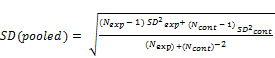(12)

(Whereandare the numbers in the experimental and control groups, respectively, andand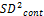are their variances.)

To calculate the effect size g and its correction d, In meta-analysis, we use the Cohen's g defined as: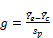(13)

Where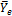is the mean of the experimental group,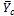is the mean of the control group, and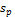is the pooled sample standard deviation, where g is a biased estimator of the population effect size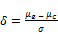(14)

According to DeCoster (2004), g can be corrected by multiplication of the term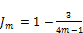(15)

where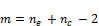The resulting statistic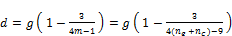(16)

is known as Hedges'd, which is an unbiased estimator ofThe variance of d, given relatively large sample, is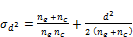(17)

The confidence level c can forbe constructed by(18)

Where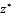is the critical value from the normal distribution.

The pooled standard deviation can be calculated from two groups by the formula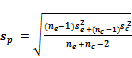(19)

Following DeCoster(2004), the t statistic for between subjects that compares the experimental and control group is given by the formula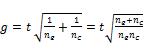(20)

when we have the same number of subjects in the experimental and control group, the above formula can be reduced to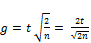(21)

Where in using z–score comparing the experimental and control groups,(22)

Whereas for F statistic comparing the experimental and control groups,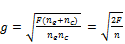(23)

The method of calculating g from within-subjects design is similar to that of between-subjects comparison. Hence, depending on the above logic,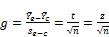(24)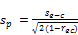(25)

where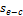is the standard deviation of the difference score and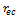is the correlation between the experimental and control scores.

Based on the above formulas values, the larger the effect size, the greater is the impact of an intervention. Cohen suggested that a correlation of 0.5 is large, 0.3 is moderate, and 0.1 is small Cohen defined.40 as the medium effect size because it was close to the average observed effect size (Aguinis, & Harden (2009)). The usual interpretation of this statement is that anything greater than 0.5 is large, 0.5-0.3 is moderate, 0.3-0.1 is small, and anything smaller than 0.1 is trivial.

3.2. Effect Size, Significance and Meta-analysis Results

Meta-analysis was invented to be a more objective way of surveying the literature on a subject. The hard work of a meta-analysis is finding all the studies and extracting the necessary information from them, so it's tempting to be impressed by a meta-analysis of a large number of studies. A meta-analysis of 50 studies sounds more impressive than a meta-analysis of 5 studies; it's 10 times as big and represents 10 times as much work, after all.

Table 1. Effect size levels for different tests.

 small medium large t-test for means d 0.20 0.50 0.80 t-test for correlation r 0.10 0.30 0.50 F-test for regression f2 0.02 0.15 0.35 F-test for ANOVA f 0.10 0.25 0.40 chi-square w 0.10 0.30 0.50

Table 2. Effect sizes for two groups.

 d r r2 f f2 2 0.707 0.49985 0.999698 0.999396 1.8 0.669 0.44756 0.900086 0.810155 1.6 0.625 0.39063 0.800641 0.641026 1.4 0.573 0.32833 0.699160 0.488824 1.2 0.514 0.26420 0.599214 0.359058 1.0 0.447 0.19981 0.499702 0.249702 0.8 0.371 0.13764 039951 0 0.159610 0.6 0.287 0.08237 0.299604 0.089763 0.4 0.196 0.03842 0.199877 0.039951 0.2 0.100 0.01000 0.100504 0.010100 0.1 0.05 0.0025 0.050063 0.002506 0 0 0 0 0

*Notice the relationship between d, r, and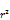.

The interpretations of effect-sizes given in Table (1), in which a suggested values for low, medium and high effects is given, depend on the assumption that both control and experimental groups have a 'normal' distribution, otherwise, it may be difficult to make a fair comparison between an effect-size based on normal distributions and one based on non-normal distributions. In practice, the values for large effects may be exceeded with values Cohen's d greater than 1.0 not uncommon. Considering table (1) and table (2), it can be noted that, d can be converted to r and vice versa. For example, the d value of 0.8 corresponds to an r value of 0.371. The square of the r-value is the percentage of variance in the dependent variable that is accounted for by the effect in the explanatory variable groups. For a d value of 0.8, the amount of variance in the dependent variable by membership in the treatment and control groups is 13.8%. T-tests are used to evaluate the null hypothesis. For this test, the effect size symbol is r. If the desired effect size is known, statistical power and needed sample size can be calculated. For instance, if the target is to find how many elements are need in a study for a medium effect size (r = 0.30) with an alpha of.05. and power of 0.95, this information can be used to find the answer.

Table 3. Effect size, Sample size &Power (Alpha=0.05; Power=0.95).*

 Effect size Delta Critical t Total sample size Actual power 0.001 3.605 1.96 51978840 0.95 0.1 3.606 1.96 5200 0.95 0.2 3.608 1.962 1302 0.95 0.3 3.613 1.964 580 0.95 0.4 3.622 1.967 328 0.951 0.5 3.623 1.971 210 0.95 0.6 3.65 1.976 148 0.952 0.7 3.671 1.982 110 0.953 0.8 3.666 1.989 84 0.952 0.9 3.711 1.997 68 0.955 10.00 10.000 4.303 4 0.993

Footnotes:*Power depends on the effect size, the sample size and the significance level.

For ANOVA, the effect size index f is used, and the effect size index from the group means can then be computed. Power is the chance that if "d" exists in the real world, one gets a statistically significant difference in the data. if the power level is taken to be 80%, there is an 80% chance to discover a really existing difference in the sample. Alpha is the chance that one would conclude that an effect difference "d", has been discovered, while in fact this difference or effect does not exist. If alpha is set at 5%, this means that in 5%, or one in twenty, the data indicate that "something" exists, while in fact it does not. In table (3), consider that: power = 1-β = p (HA is accepted/HA is true). Set α, the probability of false rejecting Ho, equal to some small value. Then, considering the alternative hypothesis HA, choose a region of rejection such that the probability of observing a sample value in that region is less than or equal to α when Ho is true. If the value of sample statistic falls within the rejection region, the decision is made to reject the null hypothesis. Typically is set at 0.05, and critical t values are specified. The calculation works as follows: Entering α=0.05, power=0.95, effect size specified as in column (1), we find the needed elements (sample size (column 4)) and so on. The effect size is seen in table (3) Column (1). The effect size conventions are small =0.20, medium=0.50, large=0.80. Calculate d and r using t values and df (separate groups t test) calculate the value of Cohen's d and the effect size correlation r, using the t test value for a between subjects t test and the degrees of freedom. Results are shown in table (4), while in table (5), d and r are calculated using t values and df.

Table 4. Calculate d and r using means and st. ds for two groups.

 Group I Group II M1 SD1 Cohen's d Effect size r M2 SD2 Cohen's d Effect size r 1 1 0 0 1 1 0 0 2 5 0.505- 0.245- 6 10 0.505- 0.245- 5 10 0.632- 0.302- 10 5 0.632- 0.302- 5 10 0.5 0.243 0 10 0.5 0.243 15 50 0.1- 0.049- 20 50 0.1- 0.049- 20 50 0 0 20 10 0 0.5 50 100 0.380 0.186 20 50 0.380 0.186 50 100 -0.280 0.139- 50 100 -0.280 0.139-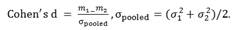Note: d and r are positive if the mean difference is in the predicted direction.

Table 5. Calculate d and r using t values and degrees of freedom.

 T value D f Cohen's d Effect size r 1 1 2 0.7071 1.5 2 2.1213 0.7276 2.0 5 1.7888 0.6666 2.0 10 1.2649 0.5345 2.5 30 0.9128 0.4152 3.0 30 1.0954 0.4803 3.0 50 0.8485 0.3905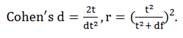Note: d and r are positive if the mean difference is in the predicted direction.

4. Discussion

Meta-analyses can play a key role in planning new studies. The meta-analysis can help identify which questions have already been answered and which remain to be answered, which outcome measures or populations are most likely to yield significant results, and which variants of the planned intervention are likely to be most powerful. Meta analysis can be used as a guide to answer the question 'does what we are doing make a difference to X? 'even if 'X' has been measured using different instruments across a range of different people. Meta-analysis provides a systematic overview of quantitative research which has examined a particular question. The appeal of meta analysis is that it in effect combines all the research on one topic into one large study with many participants. The danger is that in amalgamating a large set of different studies the construct definitions can become imprecise and the results difficult to interpret meaningfully.

Meta-analysts disagree on the criteria for inclusion or exclusion of primary studies, with relation to publication status, comparability and required scientific quality, but sensitivity analyses make it possible to assess the impact of various selection criteria on the results based on effect analysis.

Used in meta-analysis, the effect size refers to the magnitude of the effect under the alternative hypothesis. It should represent the smallest difference that would be of significance. It varies from study to study. It is also variable from one statistical procedure to the other. It could be the difference in cure rates, or a standardized mean difference or a correlation coefficient. If the effect size is increased, the type II error decreases. Power is a function of an effect size and the sample size. For a given power, 'small effects' require larger sample size than 'large effects'. Power depends on (a) the effect size, (b) the sample size, and (c) the significance level. But if the researcher knew the size of the effect, there would be no reason to conduct the research. To estimate a sample size prior to doing the research, requires the postulation of an effect size, which might be related to a correlation, an f-value, or a non-parametric test. In the procedure implemented here,'d' is the difference between two averages, or proportions. Effect size 'd' is mostly subjective, it is the difference you want to discover as a researcher or practitioner and it is a difference that you find relevant. However, if cost aspects are included,'d' can be calculated objectively. The size of the difference in the response to be detected, which relates to underlying population, not to data from sample, is of importance since it measures the distance between the null hypothesis (HO) and specific value of the alternative hypothesis (HA). A desirable effect size is the degree of deviation from the null hypotheses that is considered large enough to attract the attention. The concept of small, medium, and large effect sizes can be a reasonable starting point if you do not have more precise information. (Note that an effect size should be stated in terms of a number in the actual units of the response, not a percent change such as 5% or 10 %.).

Sample size determination and power analysis involve steps that are fundamentally the same. These include the investigation of; type of analysis and null hypothesis; power and required sample size for a reasonable range of effect as well as calculation of the sample size required to detect a reasonable effect with a reasonable level of power. Although effect size is a simple and readily interpreted measure of effectiveness, it can also be sensitive to a number of spurious influences, so some care needs to be taken in its use.

5. Conclusion

Meta-analysis should be seen as structuring the processes through which a thorough review of previous research is carried out. The issues of completeness and combinability of evidence, which need to be considered in any review are made explicit. On the use of Meta-analysis, the following can be summarized:

i.  Despite limitations, meta-analytic approaches have demonstrable benefits in addressing the limitations of study size, can include diverse populations, provide the opportunity to evaluate new hypotheses, and are more valuable than any single study contributing to the analysis.

ii.  Assumptions about the population nature is essential in using effect size, for the interpretation depends mainly on the assumptions of normality and equality of deviations of 'control' and 'experimental' group values. Effect sizes can be interpreted in terms of the percentiles or ranks at which two distributions overlap.

iii.  Use of an effect size with a confidence interval holds the same information as a test of statistical significance, but with the emphasis on the significance of the effect, rather than the sample size.

iv.  Like all types of research, meta-analyses has both potential strengths and weaknesses. meta-analysis does not work nearly as well as we might want it to work. The problems are so deep and so numerous that the results are simply not reliable. Meta-analysis simply does not work very well in practice.

v.  Meta-analysis is superior to narrative reports for systematic reviews of the literature, but its quantitative results should be interpreted with caution even when the analysis is performed according to rigorous rules.

vi.  By using meta-analysis, a wide variety of questions can be investigated, as long as a reasonable body of primary research studies exist.

References

1. Aguinis,H.& Haren,E.E.(2009).Sample size rules of thumb: Evaluating three common practices. In Charles E. Lance and Robert J.Vandenberg (Eds.),Statistical and methodological myths and urban legends: Doctrine, verify and fable in the organizational and social sciences (pp.267-286).NY York:Routledge.
2. Baker,R.,&Dwyer,F.(2000).A meta-analytic assessment of the effects of visualized instruction, paper presented at the 2000 AECT National Convention. Long Beach,CA.
3. Becker,L.,(2000). Effect Size (ES), This website provides an overview about effect size and its measures.
4. Berman, N.G., and R.A. Parker.(2002). Meta-analysis: neither quick nor easy. BMC Medical Research Methods 2:10.
5. Biostat (2006).Meta-analysis. [On-line] available: http://www.meta-analysis.com/.
6. Bushman, B. J., & Wells, G. L. (2001). Narrative impressions of literature: The availability bias and the corrective properties of meta-analytic approaches. Personality and Social Psychology Bulletin, 27, 1123-1130.
7. Buchner,A, Erdfelder,E. and Faul,F. How to use G*power. Viewed 19 Jun 2009
http://www.psycho.uni-duesseldorf.de/aap/projects/gpower/how_to_use_gpower.htm.
8. Cohen, J. (1988). Statistical power analysis for the behavioral sciences (2nd ed.). Hillsdale, NJ: Lawrence Earlbaum Associates.
9. DeCoster,J.(2004). Meta-analysis Notes. Retrieved <month, day, and year you downloaded this ¯le>from http://www.stat-help.com/notes.html.
10. Egger M, Davey Smith G, Schneider M, Minder C(1997). Bias in meta-analysis detected by a simple, graphical test. BMJ 1997;315:629-34.
11. Gerard E. Dallal(2015). Meta Analysis. The Little Handbook of Statistical Practice BMJ 2003;327:557-560 (6 September).Copyright © 2003 Gerard E. Dallal Last modified: 07/28/2015 17:39:27.
12. Glass,G.V.(1976). Primary, secondary, and meta-analysis of research. Educational Researcher, 5, 3-8.
13. Glass, G.V.(1977). Integrating findings: The meta-analysis of research. Review of Research in Education, 5, 351-379.
14. Gurevitch, J., and L.V. Hedges. (2001).Meta-analysis: combining the results of independent experiments. pp. 347-369 in Design and Analysis of Ecological Experiments, S.M. Scheiner and J. Gurevitch, eds. Oxford University Press,
15. Haidich A,B.Hippokratia.(2010).Dec; 14(Suppl 1): 29–37.PMCID: PMC3049418Meta-analysis in medical research.
16. Hedges, L.V., and I.Olkin. (1985). Statistical methods for meta-analysis. Academic Press, London.
17. Huberty, C.J. (2002) 'A history of effect size indices'. Educational and Psychological Measurement, 62, 2, 227-240.
18. James Neil(2006). Meta-analysis Research Methodology. Quantitative Research Methods Last updated:10 May 2006.
19. J Hypertens Suppl. 1996 Sep;14(2):S9-12; discussion S13.Advantages and disadvantages of the meta-analysisapproach.US National Library of Medicine National Institutes of HealthPubMed.
20. Jonathan J Deeks, Julian PT Higgins and Douglas G Altman on behalf of the Cochrane Statistical Methods Group.Analyzing data and undertaking meta-analyses, Part 2: General methods for Cochrane reviews.
21. McDonald, J.H.(2014).Handbook of Biological Statistics (3rded.).Baltimore,Maryland.http://www.biostathandbook.com/metaanalysis.html. revised December 4, 2014.
22. Mike Fur(2008).Effect sizes and significance Tests.Psychology Dept. Wake Forest university (March,2008).
23. Morris, S. B., & DeShon, R. P. (2002). Combining effect size estimates in meta-analysis with repeated measures and independent-groups designs. Psychological Methods, 7, 105-125.
24. National Center for Biotechnology Information, U.S. National Library of Medicine8600 Rockville Pike, Bethesda MD, 20894 USA.
25. Olejnik, S. and Algina, J. (2000).Measures of Effect Size for Comparative Studies: Applications, Interpretations and Limitations. Contemporary Educational Psychology, 25, 241-286.
26. Overview of Meta-Analysis | Books on Meta-Analysis | Other Meta-Analysis Sites | Publication Bias.
27. Poston,J.M,&Hanson,W.E.(2010).Meta-analysis of psychological assessment as a therapeutic intervention. Psychological Assessment,22,203-12.
28. Richard A.Zeller and Yan(2007).Establishing the Optimum Sample Size.Epidemiology and Medical Statistics,Handbook of Statistics Vol 27,Pages 656-678.
29. Rosenthal, R. (1994) 'Parametric Measures of Effect Size' in H. Cooper and L.V. Hedges (Eds.), The Handbook of Research Synthesis. New York: Russell Sage Foundation.
30. Rosenthal, R.Rosnow,R.L.&Rubin (2000)D.B. Contrasts and Effect sizes in behavioral research:A correlational approach. Cambridge.UK. Cambridge University Press.
31. Rudner, Glass, Evartt, & Emery (2002). A user's guide to the meta-analysis of research studies.
32. Seto KC, Fragkias M, Güneralp B, Reilly MK (2011) A Meta-Analysis of Global Urban Land Expansion. PLoS ONE 6(8): e23777. doi:10.1371/journal.pone.0023777.
33. Slavin,R.,&Smith,D.(2008).Effects of sample size on effect size in systematic reviews in education.Paper presented at the annual meetings of the Society for Research on Effective Education, Crystal City,VI.
35. Wolf, F. M. (1986). Meta-analysis: Quantitative methods for research synthesis. Beverly Hills, CA: Sage.

BiographyDr. habib Ahmed Elsayir received his Ph. D degree in Statistics from Omdurman Islamic University, Sudan in 2001. He was appointed manager of Omdurman Islamic University Branch at AlDaein 2002-2005. Now he is associate prof in the Dept. of Mathematics, Al qunfudha University college, University of um Al Qura, Saudi Arabia.
 Contents 1. 1.1. 1.2. 2. 2.1. 2.2. 2.3. 3. 3.1. 3.2. 4. 5.
Article ToolsAbstractPDF(328K)